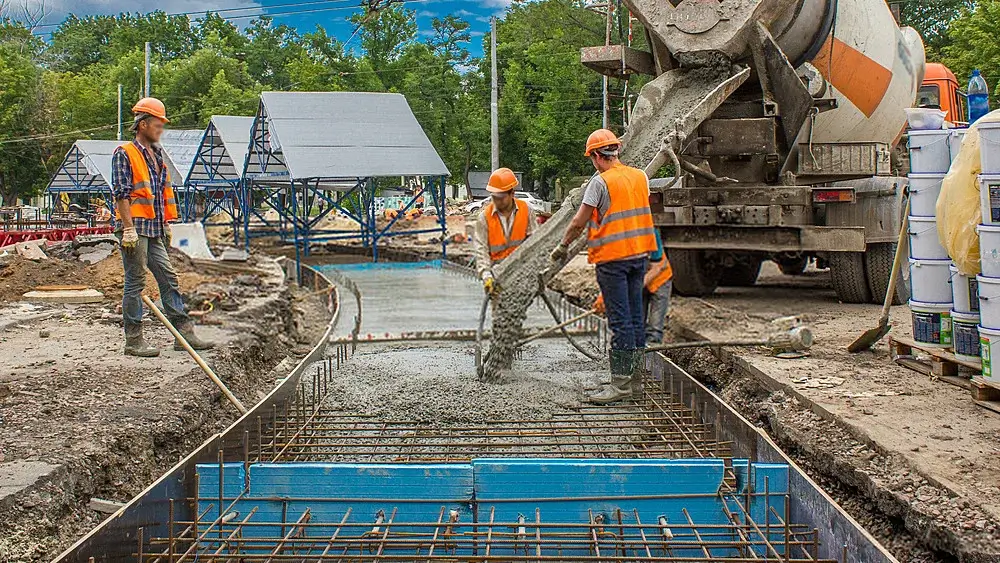Quantities of components for concrete likewise cement, aggregates, gravel, and sand for the development of the needed amount of concrete of offered mixed rations like 1:2:4 (M15), 1:1.5: 3 (M20), 1:1:2 (M25) could be measured by accurate volume method. This method has been dependent on the standard of utterly compressed volume methods that is similar to the perfect volume of all concrete materials. For calculating concrete, the structure might be based on beams, columns, slabs, and foundations, which has been dependent on the kind of structure. The volume of concrete needed for the framework could be measured by merging up the books of each operational member or every part of the members.

## Calculating How Much Concrete Is Needed

As we all know, concrete is the mixture of gravel, water, sand, and cement, which is sold by the cubic yard most of the time, but it is also available in the form of Ready mix concrete bags. It is significant to measure the amount of material required as ideally as probable since you frequently only obtain one chance for the pour. So how much concrete is needed? Here the whole procedure is covered for approximating concrete in detail discussed below. Still, you can also utilize the calculator by using the following steps:

## Steps For Calculating Concrete Requirements For Your Project

The easy and simple steps to determine the amount of cement mixture for your project are defined below

• Find out how much thickness you would require
• Calculating concrete volume length and width of the spaces which you want to cover
• Determine the height and width of the product for finding out the square foot
• Now transform the density from inches to feet
• Measure the width of concrete in feet and square feet for cubic feet results
• Then exchange cubic feet to cubic yards by multiplying with 0.037

### Step 2: Measure all the dimensions of the site.

To evaluate the site’s dimensions, you need to divide the general cubic yards by revenue. You can use the following yields as per the proportions of the size of the bag

• 40-pound bag yields have 0.011 cubic yards
• The 60-pound bag could cover 0.017 yards
• The 80-pound bag could crop 0.022 cubic yields

### The calculation for cement quantity

Cement= (1/5.5) × 1.54=0.28m3 , in this 1 s taken as the part of cement, 5.5 is the figure of proportion, and the density of cement is 1440/m3= 0.28×1440=403.2 kg

We also know that every bag of cement is= 1.226 CFT

To measure in Cubic Feet= 8×1.225=9.8 cubic feet

#### Calculation for sand

Contemplate volume for concrete=1m3

Dry volume of concrete= 1×1.54 = 1.54=1m3

Sand= (1.5/5.5)×1.541m3

Density of sand is 1450/m3

sand=0.42×1450= 609kg

As we know that 1m3= 35.31 CFT

For calculation in Cubic Feet= 0.42×35.31= 14.83 CB

#### Calculation for Aggregate quantity

Consider volume of concrete=1m^3

Dry volume of concrete=1×1.54=1.54m^3, 3 is the part of cement, 5.5 is the total ratio.

Density of Aggregate is 1500/m^3

Calculation for kg= 0.84×1500= 1260 kg

As we know that 1m^3= 35.31= 29.66 Cubic Feet

### Step 4: Receive a cost estimate based on the substantial quantity required.

Concrete = 1500 kg/m3 = 93.642 lb/ft3 = 2528.332 lb/cubic yards Sand = 1700 kg/m3 = 105 lb/ft3 = 2865.443 lb/cubic yards Coarse totals = 1650 kg/m3 = 103 lb/ft3 = 2781.166 lb/cubic yards Specific gravities of substantial materials are as per the following: Cement = 3.15 Sand = 2.6 Coarse totals = 2.6. The level of entrained air accepted is 2%. The blend extent of 1:1.5:3 by dry volume of materials can be communicated as far as masses as: Cement = 1 x 1500 = 1500 Sand = 1.5 x 1700 = 2550 Coarse total = 3 x 1650 = 4950.